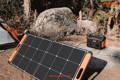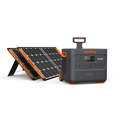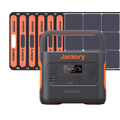## The Ultimate Guide to Volts to Watts Conversion

When buying a solar generator or portable power station, you'll first come across two crucial electrical rating terms — watts and volts. Calculating watts of specific home appliances will help you select a solar generator that generates appropriate power output to keep devices running for long hours.

However, sometimes the power output is given in volts. That's where volts to watts conversion comes in. If you know the volts of an appliance, you can quickly convert them to watts using the formula: P = V × I.

Jackery Solar Generators come in different sizes to meet all the power needs of customers. For instance, if you want a solar generator for off-grid living, you can consider Jackery Solar Generator 2000 Pro.Whether you're a homesteader, an off-grid adventurer, or just want a reliable backup power source, this ultimate guide will help you make sense of the numbers and choose the right size solar generator.

## Volts To Watts

Volts to watts conversion is necessary as it helps you understand how much power an electrical device or appliance consumes. Once you know the wattage of all the home electrical appliances, you can combine them to find the right size of inverter.

Volt is the unit of measure of the electric force responsible for the flow of an electric circuit. One volt is the amount of pressure/force required to flow one ampere current against one ohm of resistance.

On the other hand, watt measures the amount of power consumed by any electrical appliance. It also helps you select a power generator that meets your energy needs. Below we have explained volts and watts and why these electrical ratings are essential in solar power.

### What Is Volt?

A volt is a measure of voltage and represents the potential difference between two points of a conducting wire. It is the speed of electrons when they pass through a specific location in a circuit. One volt is equal to 0.001 kilowatts (kW) or 1000 watts (W) per hour.

If you know the voltage of a device, you can quickly determine the amount of current it draws. Calculating the watts of an appliance will help you figure out how much power will be drawn through any circuit by plugging it into a charging device.

In the solar power system, the solar panels generate electrical energy that can be measured in volts. Understanding volts helps you find a compatible solar system that will charge all appliances efficiently.

### What Is A Watt?

Wattage, in simple words, is the rate of energy used by a device. The unit of power helps us determine how much energy is needed to perform work or generate heat. One watt is equivalent to one joule per second, where a joule indicates a measure of power.

The power output of the solar panels is measured in watts. It represents the maximum amount of energy it can generally produce under ideal conditions, such as when the sun shines directly on the panel at peak intensity.

You can calculate the total power output of a solar power system from the number and size of the solar panels and the amount of peak solar energy that is available.

### Why Are Electrical Ratings Important In Solar Power?

Electrical ratings, including current, voltage and power, are essential when determining the right solar-based system. You'll need to consider the electrical ratings of solar panels, inverters, and battery storage to identify which generator meets your power needs and can deliver power for long hours.

The three electrical ratings – volts, amps, and watts – are interrelated. For instance, if you've two of them, you can easily find the third one using a simple formula or with a watts to volts calculator.

## How To Convert Volts To Watts?

Now that you know what watts and volts are, it's time to understand how to convert them.

Simply put, you can use Watt's Law to convert volts to watts. The law states current is equivalent to the power generated divided by the voltage.

The simple formula to convert volts to watts include:

P (Watts) = A (Ampere) × V (Volts)

Where,

P = Power

A = Current in amps

V = Voltage

### Volts To Watts Conversion Formula

Below we have mentioned some simple formulas that will help you convert volts to watts.

Formula 1: To Convert DC Volts to Watts

The power of an electrical device is equal to voltage times the current if it is a DC circuit.

P (in Watts) = V (in Volts) × I (in Ampere)

Formula 2: To Convert AC Single Phase Volts to Watts

The power is equal to PF (power factor) times the RMS voltage times the phase current in the ampere.

P (in Watts) = PF × I (in Amps) × V (in Volts)

Formula 3: To Convert Volts to Watts using Resistance

Sometimes, you know the resistance of the circuit but not the ampere value. In this case, you can use the below-mentioned formula to convert volts to watts.

P (in Watts) = (V)² ÷ R (in Ohm)

### Volts To Watts Conversion Examples

Let us take three simple examples to help you understand how to use these conversion formulas.

Formula 1: P (in Watts) = V (in Volts) × I (in Ampere)

Example: Suppose you need to convert 120 volts to watts for a DC circuit having 2 amperes of current.

P = 120 V × 2 A = 240 Watts.

So, 120 volts will consume approximately 240 watts of power, given that the amp is 2A.

Formula 2: P (in Watts) = PF × I (in Amps) × V (in Volts)

Example: Suppose you want to convert 240 volts to watts for an AC electric circuit having 10 amps of current and a power factor of 0.9.

P = 240 V × 10 A × 0.9 = 2,160 watts.

So, 240 volts will consume 2,160 watts of power, given that PF is 0.9 and amps are 10.

Formula 3: P (in Watts) = (V)² ÷ R (in Ohms)

Example: Suppose you want to convert 12 volts to watts for a DC circuit having 6 ohms of resistance.

P = (12V)² ÷ 6 Ω = 144 V² ÷ 6 Ω = 24 Watts.

### Volts To Watts Conversion Chart

Based on the formula, here are the volts to watts conversion chart.

Volts (V)

Amp (A)

Watts (W)

How many watts in 10 volts?

1 amp

10 watts

How many watts in 20 volts?

1 amp

20 watts

How many watts in 30 volts?

1 amp

30 watts

How many watts in 40 volts?

1 amp

40 watts

How many watts in 50 volts?

1 amp

50 watts

## How To Convert Watts To Volts?

Like volt to watt conversion, you can convert watts to volts using the Watt's Law power formula. It states that current is equal to power divided by voltage.
Here is the mathematical representation of the conversion:
V (in Volts) = P (in Watts) ÷ I (in Ampere)
Where,
V = Voltage
P = Power
A = Current in amps

### Watts To Volts Conversion Formulas

To calculate the volts, here are a few simple formulas.
Formula 1: To Convert DC Watts to Volts
The voltage of the electrical device is equal to the power in watts divided by the circuit's current in amperes.
V (in Volts) = P (in Watts) ÷ I (in Ampere)
Formula 2: To Convert Watts to Volts in an AC Circuit
Unlike DC circuits, you'll need to consider the AC circuit's power factor (PF) during conversion.
V (in Volts) = P (in Watts) ÷ [I (in Amps) × PF]
Formula 3: To Convert Watts to Volts using Resistance
If the resistance of the circuit is known, you can easily convert watts to volts using the below-mentioned formula.
V (in Volts) = √[P (in watts) × R (in Ohms)]

### Watts To Volts Conversion Examples

Different formulas to convert watts to volts can be applied in different scenarios. For instance, if you know the resistance, you can use formula 3. Alternatively, if you know the ampere, you can choose the formula 1 or 2 depending upon the type of current.
Formula 1: V (in Volts) = P (in Watts) ÷ I (in Ampere)
Example: Suppose you want to convert 30 watts to volts using a DC circuit with 5 amps of current.
V (in Volts) = 30 watts ÷ 5 amps = 6 volts.
Formula 2: V (in Volts) = P (in Watts) ÷ [I (in Amps) × PF]
Example: Suppose for an AC circuit with a power factor of 0.9 and 12 amps, you want to convert 1400 watts into volts.
V (in Volts) = 1400 ÷ [12 × 0.9] = 129.6 volts.
Formula 3: V (in Volts) = √[P (in watts) × R (in Ohms)]
Example: If the resistance of the circuit is 20, you can convert 125 watts into volts using this simple formula.
V (in Volts) = √(125 W × 20 Ω) = √2500 = 50 volts.

### Watts To Volts Conversion Chart

Here are a few watts to volts direct conversions.

Watts (W)

Amps (A)

Volts (V)

How many volts in 10 watts?

1 amp

10 volts

How many volts in 20 watts?

1 amp

20 volts

How many volts in 30 watts?

1 amp

30 volts

How many volts in 40 watts?

1 amp

40 volts

How many volts in 50 watts?

1 amp

50 volts

## Volt Vs. Watts: What Differences

Here is the breakdown of critical differences between volts and watts.

Comparison

Watts (W)

Volt (V)

Definition

It is the SI unit of power.

It is the SI unit of EMF and potential difference.

Units of

Power

Potential difference and EMF

Symbol

W

V

Measurement

It measures realistic power.

It measures a small amount of voltage from power.

Difficult

Easy

Measuring device

Power Meter

Voltmeter

Base unit

Kg M^2 A^-1 S^−3

Kg M^2 S^−3

Formula

Watts = Volts × Current

Volt = Potential Energy ÷ Charge

One of the major differences between watts and volts is the former measures the SI unit of power. On the other hand, a volt is the SI unit of electromotive force and potential difference. Some other differences between the two include the following:
Voltage (measured in volts) represents the potential difference between two points, whereas power (measured in watts) defines the rate at which energy is transferred.
Watts are often related to electrical flow, whereas voltage is connected to electrical pressure.
A voltmeter is used to measure the volts; meanwhile, a power meter can measure watts.

## Best Solar Generator With JackeryJackery is a well-known brand offering affordable and powerful solar generators. The generators convert the sun's light to electrical energy. It consists of two main components: portable solar panels and a power station.
Jackery SolarSaga Solar Panels capture the sun's light that falls on the photovoltaic cells. Meanwhile, the Jackery Explorer Portable Power Station stores the electrical power generated. You can take your portable solar generator to charge all your electrical devices wherever you go.
Jackery offers various solar generators to cater to users with different budgets and power needs. The top two Jackery Solar Generators include:

### Jackery Solar Generator 2000 Pro

The Jackery Solar Generator 2000 Pro is the best power source for outdoor and camping enthusiasts. The best part about the portable solar generator is that it takes only 2.5 hours to charge and weighs only 19.5 kg. You can take the solar panels and the portable power station anywhere and everywhere. It can charge almost 99% of appliances and keep them running for hours.

Capacity

Recharging Time

Ports

Appliances

2160Wh (43.2V/50Ah)

Explorer 2000 Pro + 6*SolarSaga 100W: 5.5H

2*AC Output: 230V, 2200W (peak 4400W); 2*USB-A: Quick Charge 3.0x2, 18W Max; 2*USB-C: 100W Max, (5V, 9V, 15V, 12V, 20V up to 5A); 1*Carport: 12V⎓10A

TV(200W): 8.4H

Refrigerator(700W): 2.4H

Microwave(750W): 2.2H

Coffee Maker(800W): 2.1H

Blender(300W): 5.6H

Stove(850W): 2H

### Jackery Solar Generator 1000 Pro

With a battery capacity of 1002 Wh, Jackery Solar Generator 1000 Pro is a powerful and portable solar system. It can help you power all your devices, including but not limited to lights, laptops, phones, and mini-fridges. As it is designed with industrial-grade components, Jackery Solar Generator 1000 Pro is a reliable energy source with a super long lifespan.Capacity

Recharging Time

Ports

Appliances

1002Wh (43.2V, 23.2Ah)

Explorer 1000 Pro + 6*SolarSaga 80W: 9H

2*AC Output: 230V, 1000W, peak 2000W; 2*USB-A: Quick Charge 3.0x2, 18W Max; 2*USB-C: 100W Max, (5V, 9V, 15V, 12V, 20V up to 5A); 1*Carport: 12V⎓10A

TV(200W): 4H

Fridge(500W): 1.6H

Blender(300W): 2.7H

CPAP Machine(100W): 8H

Computer(200W): 4H

Electric Wheelchair(380W): 2.1H

## Volts To Watts FAQs

Below are a few frequently asked questions regarding volts to watts conversion.

1. How many watts are 120 volts?

To calculate the watts, you must apply the formula P = V × I. As the current (I) flowing through the circuit is not defined, you can't use the formula directly.

Additionally, it's worth noting watts will depend on the flow of electric charge. You'll need to consider the power factor PF to convert 120 volts into watts in an AC circuit.

But if it's a DC current, you can directly substitute the values in the given formula.

Here's an example:

Suppose you want to convert 120 volts to watts where the power factor is 0.9, and the electric flow of current is 2 amperes.

For direct current:

Power = 120 V × 2 A = 240 watts.

For alternating current:

Power = 120 V × 2 A × 0.9 = 216 watts.

Note: These values will differ when the power factor and ampere current is altered.

2. How many watts are 240 volts?

Like how we converted 120 volts to watts, you can convert 240 volts.

Suppose the power factor and ampere in the case of a DC circuit are 0.9 and 2 A, respectively.

Here is how you can calculate it:

For direct current:

Power = 2 A × 240 V = 480 watts.

For alternating current:

Power = 2 A × 240 V × 0.9 = 432 watts.

3. Why convert volts to watts?

Converting volts to watts is vital as it allows you to understand the power consumption of a particular electrical device. Additionally, it assists you in choosing the right solar generator size to support your power needs.

3. How many watts does a solar generator produce?

Solar generators provide renewable energy whenever and wherever you need it. However, different solar generators have different capacities and watts. That's why Jackery offers solar generators equipped with power stations of various sizes.

Here are the two best Jackery Solar Generators with their capacities:

Jackery Solar Generator 2000 Pro can provide 2200-watt output power and 4400-watt peak surge power. It is equipped with a 2160 Wh charging capacity.

Jackery Solar Generator 1000 Pro has a battery capacity of 1002Wh with a peak surge power of 2000 watts.

All the Jackery solar generators are portable and safe to use indoors as they emit no toxic fumes. You can charge most of your home electrical appliances with these powerful and portable solar generators.## Final Thoughts

Volts and watts are critical for an electric appliance or a solar system. You can purchase a compatible solar generator by understanding how many watts an electrical device uses.

Now that you know the easy formula to convert volts to watts, it's time to calculate the power usage of all electrical devices. Once you know your power needs, you can choose Jackery Solar Generators in different sizes.

If you are perplexed about which solar generator to choose, you can consider Jackery Solar Generator 2000 Pro, which features fast charging and high battery capacity.

### New Arrival

Hurry up! Sale ends once the timer hits zero

00Days
00Hrs
00Mins
00Secs

### Best-selling Jackery Solar Generators

Sale Off### Jackery Solar Generator 2000 Plus

\$4,199.00\$3,989.00
Sale Off### Jackery Solar Generator 2000 Pro

\$5,879.00
Sale Off### Jackery Solar Generator 1000 Pro

\$3,835.00\$2,269.00